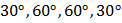# Following figure shows the multiple reflections of a light ray along a glass corridor where the walls are either parallel or perpendicular to one another. If the angle of incidence at pointis, what are the angles of reflection of the light ray at pointsandrespectivelya)b)c)d)## Question ID - 152598 :- Following figure shows the multiple reflections of a light ray along a glass corridor where the walls are either parallel or perpendicular to one another. If the angle of incidence at pointis, what are the angles of reflection of the light ray at pointsandrespectivelya)b)c)d)3537

(c)Next Question :
 The focal lengths of the objective and the eye-piece of a compound microscope areandrespectively. The distance between the objective and the eye-piece is. The final image formed by the eye-piece is at infinity. The two lenses are thin. The distances inof the object and the image produced by the objective measured from the objective lens are respectively a) 2.4 and 12.0 b) 2.4 and 15.0 c) 2.3 and 12.0 d) 2.3 and 3.0